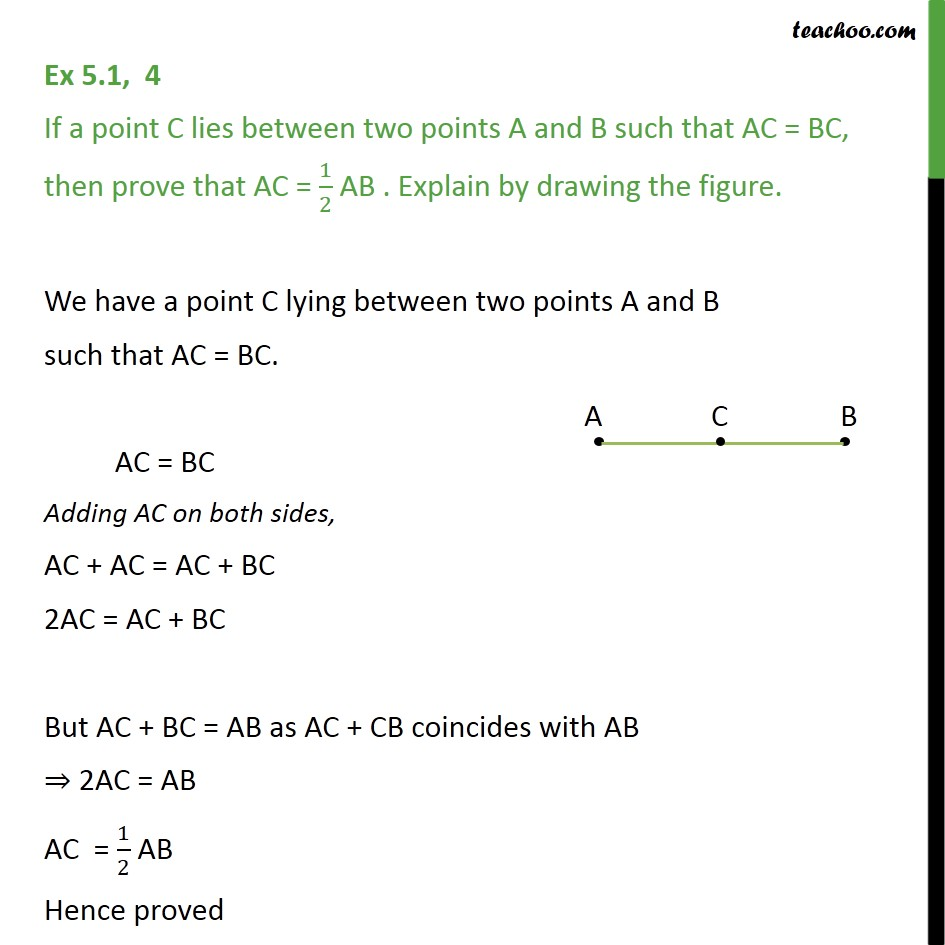Ex 5.1

Chapter 5 Class 9 Introduction to Euclid's Geometry
Serial order wiseMaths Crash Course - Live lectures + all videos + Real time Doubt solving!

### Transcript

Ex 5.1, 4 If a point C lies between two points A and B such that AC = BC, then prove that AC = 1/2 AB . Explain by drawing the figure. We have a point C lying between two points A and B such that AC = BC. AC = BC Adding AC on both sides, AC + AC = AC + BC 2AC = AC + BC But AC + BC = AB as AC + CB coincides with AB ⇒ 2AC = AB AC = 1/2 AB Hence proved